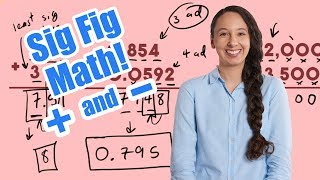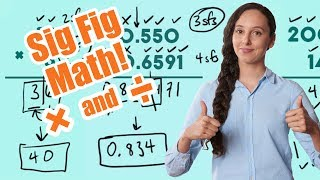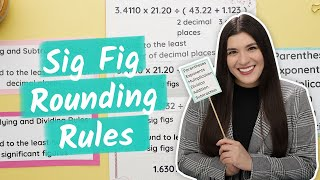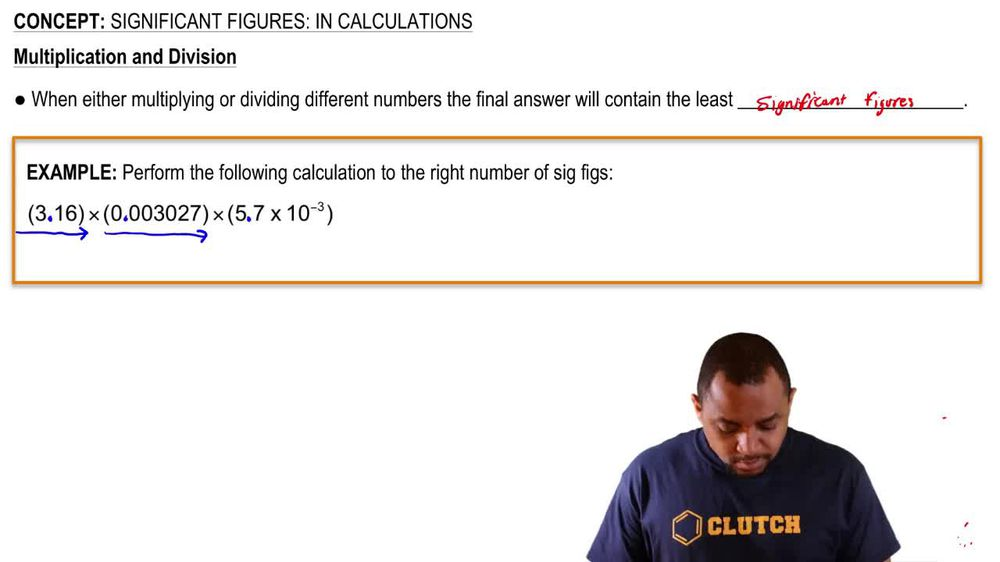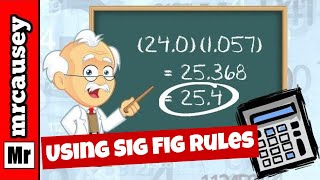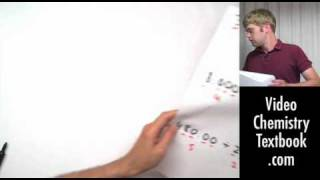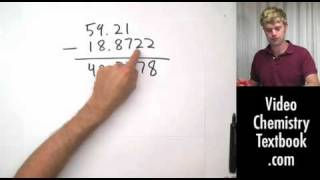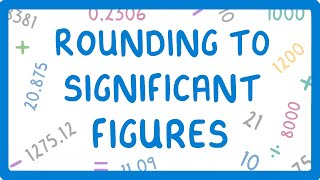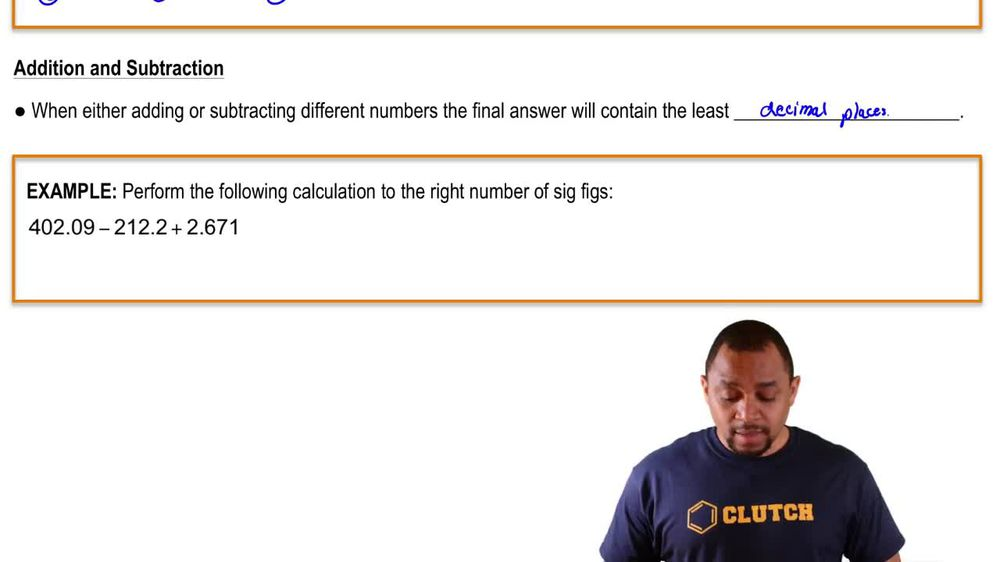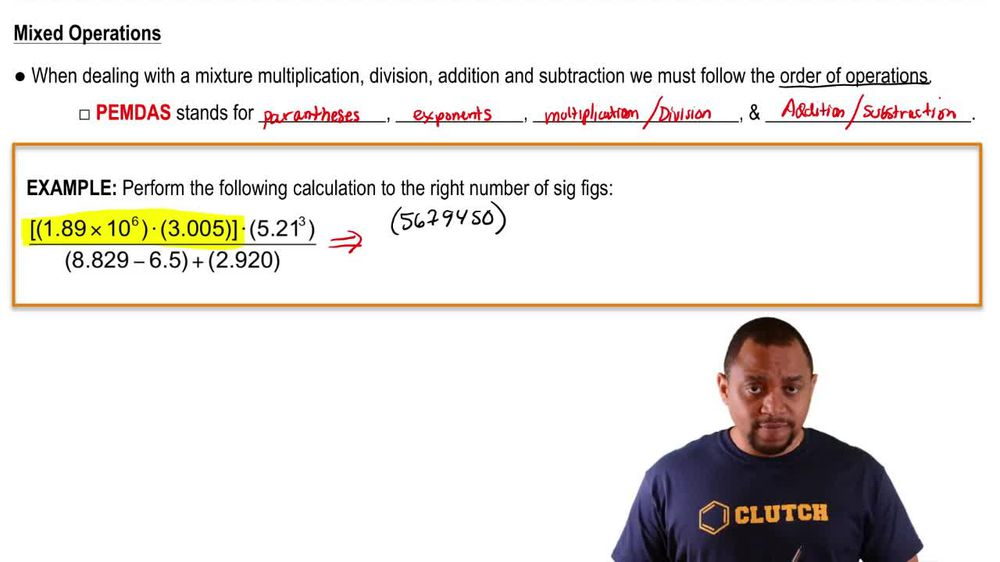Start typing, then use the up and down arrows to select an option from the list.
1. 1. Intro to General Chemistry2. Significant Figures: In Calculations
Problem

# Express the results of the following calculations with the correct number of significant figures. (a) 4.884 * 2.05

Relevant Solution1m
Play a video:
Hi everyone today we have a question asking us to form the following operation and we need to express the answer using the correct number of significant figures. So we have 7.187 times 37 times 0.009 And that equals 2.393271. Now we are multiplying and we have to remember that for multiplication and division. The number with the least number of significant figures determines our answer. So in that case, looking at our problem, that would be 0.009 with only one significant figure. So now we're looking at R2 here And we need to look at the right of R2 to decide if we need to round up or not. Now, if the number to the right of R2 is five or larger, we need to round up in this case it is not. So there's no need to round up and our answer is to thank you for watching. Bye.